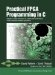# Section 7.1. A Model of FPGA Computation

### 7.1. A Model of FPGA Computation

Before we discuss specific techniques for extracting parallelism at the level of instructionsblocks of C statementswithin a process, let's consider how computation is actually performed on an FPGA. This will help you understand the hardware execution model of your C code running in hardware. If you can understand the basic architecture of the modern CPU, it's not difficult to understand the hardware execution model. As in a traditional CPU, there must be some control logic, one or more arithmetic units, and a governing clock.

Actual computation on an FPGA is done by some number of predesigned arithmetic units such as adders, subtractors, and multipliers. As in a CPU, multiple instances of these units can operate in parallel, but in the FPGA the level of parallelism (the potential number of such computational units) is limited only by the amount of available hardware and its interconnect structure.

In both the CPU and the FPGA, a clock controls the speed of operation of the arithmetic units. Each unit has one specific task to perform in each cycle. As a result, the maximum clock speed is limited by the speed of the basic arithmetic units. A CPU typically has a single clock controlling a relatively small number of such arithmetic units, while in the FPGA multiple clocks could be controlling different arithmetic units operating at different rates.

Finally, as in a CPU, an FPGA computing model must include a controller that maintains the order of execution, indicating what data should be supplied to each of the arithmetic units and where the results should go. But unlike in a CPU, the controller can (and should) be specific to the algorithm being performed.

Given that the structure of the generated hardware is much like that of a CPU, where does the speedup in computation come from? As it turns out, a number of limitations inherent in modern CPUs can be avoided by a generated model of computation.

First, a traditional CPU does not perform control and computation at the same time; that is, the CPU must spend noncomputational time decoding instructions, performing branches, and so on. In the case of FPGA-based computation these operations can be performed by a dedicated controller that operates in parallel with the arithmetic units. (It should be pointed out, however, that modern high-performance CPUs do introduce a great deal of parallelismfor example, through the use of instruction pipelining and prefetch operations.)

Second, by compiling a software process directly to hardware, it is in theory possible to generate any number of arithmetic units as needed, whereas the general-purpose CPU has only a fixed set of units from which to choose. (This fact alone has the potential to dramatically increase the processing efficiency of FPGA-based computations over a traditional processor, in which only a small fraction of the die area is actually engaged in computations at any given time.)

Third, the generated hardware may include custom single-cycle operations, which may be formed from multiple distinct statement lines in the original algorithm description, and highly optimized instruction pipelines.

Finally, a CPU generally has one connected memory resource, whereas the generated hardware for an FPGA implementation may take advantage of multiple blocks of RAM and other external resources to support multiple memory operations that are performed in parallel with each other and with other I/O operations.Practical FPGA Programming in C
ISBN: 0131543180
EAN: 2147483647
Year: 2005
Pages: 208

Similar book on Amazon